# Bearing fault diagnosis using a novel coding-statistic feature combined with NNC

## Mingquan Qiu1, Zebo Zhao2

1, 2School of Mechatronics Engineering, Guizhou Minzu University, Guiyang, P. R. China

1Corresponding author

Journal of Vibroengineering, Vol. 24, Issue 5, 2022, p. 848-861. https://doi.org/10.21595/jve.2022.22334
Received 8 December 2021; received in revised form 7 March 2022; accepted 10 April 2022; published 15 August 2022

Copyright © 2022 Mingquan Qiu, et al. This is an open access article distributed under the Creative Commons Attribution License, which permits unrestricted use, distribution, and reproduction in any medium, provided the original work is properly cited.
Views 124
Abstract.

The failures of rolling bearings usually cause the breakdown of rotating machinery. Therefore, bearing fault diagnosis is receiving more and more attentions. In this paper, a new coding-statistic feature is proposed for bearing fault diagnosis. Firstly, a waveform coding matrix (WCM) is drawn from each signal using a coding algorithm then a statistical feature is extracted from the WCM with a pre-defined dictionary. Secondly, all statistical features are processed using two-dimensional principal component analysis (2DPCA) to reduce redundant information and dimensionality. Finally, a nearest neighbor classifier (NNC) is employed to classify the bearing faults. Two bearing fault classification problems are utilized to demonstrate the effectiveness of the proposed scheme. Experimental results show that an excellent performance could be accomplished with the proposed scheme.

Highlights
• Using a pre-defined dictionary, a novel coding-statistic feature is extracted from the waveform coding matrix, which is drawn from the time-domain signal using a coding algorithm.
• All coding-statistic features are processed using two-dimensional principal component analysis to reduce redundant information then the bearing faults are classified with a simple nearest neighbor classifier.
• The effectiveness of the proposed method is demonstrated by two fault classification problems using bearing fault datasets.

Keywords: bearing, fault diagnosis, waveform coding, coding-statistic feature.

#### 1. Introduction

Rolling bearings, as the extremely essential support components in rotating machinery, are most widely used in industrial machines. Their failures may generally result in machine breakdowns, and even casualties . Hence, it is essential to diagnose their faults accurately and rapidly, especially for industrial site.

In general, three procedures are commonly included in bearing fault diagnosis. The first is to collect monitoring data with sensors. The second is to process the acquired signals to extract sensitive features related to the bearing health state. And the third is to diagnose bearing health conditions and/or locate the corresponding faults. The aforementioned fault features are usually extracted from raw signals in time domain, frequency domain and time-frequency domain. Time domain analysis is directly analyzed base on the time waveform of the vibration signals to extract features such as root-mean-square amplitude, skewness, kurtosis etc. . Frequency domain analysis is often conducted with transforming vibration signals into frequency domain via fast Fourier transform to extract features such as cepstrum, Hilbert envelope spectrum analysis etc. , . Time-frequency features are usually abstracted by means of short time Fourier transform , empirical mode decomposition , Wigner-Ville distribution , wavelet analysis , HHT time-frequency analysis, and high order spectral analysis . However, the efficiency of the extraction process is of great importance for real-time diagnosis. Hence, more attentions should be focused on the phase of feature extraction for fault diagnosis.

Once the characteristics are extracted, bearing health state can be identified with assistance from one or more classifiers, such as distance classifiers, artificial neural networks (ANNs), support vector machines (SVMs) and so on. For example, Liu et al.  performed bearing fault diagnosis with LS-SVM and Empirical Mode Decomposition. Yang et al  conducted fault diagnosis of rolling bearing based on SVMs and fractal dimension. Sreejith et al.  employed time-domain features and neural networks for bearing fault diagnosis. And deep learning algorithm are utilized for fault diagnosis of rotating machine for the past few years , . In general, artificial intelligence (AI) approaches, like ANNs and SVMs, may show an improved performance over other approaches. But in practice, however, it’s not easy to apply AI techniques to provide effective decision support due to their expensive computations. Considered their shortcomings, a simple classifier, nearest neighbor classifier (NNC), is employed in this study to classify the health states of bearings.

In this paper, a novel feature extraction method is proposed based on a coding strategy. First, a waveform coding matrix (WCM) is drawn from the time discrete series using a coding technique to capture the signal structures, and a coding-statistic feature is acquired from WCM with a pre-defined dictionary. Then two-dimensional principal component analysis (2DPCA) is employed to process these statistical features so that the redundancy and dimensionality of raw features could be rejected and reduced. Finally, an NNC is applied to classify the bearing faults. The main contributions of this work are to propose a new feature derived directly from time domain signal to interpret the health state of rolling bearings, and to perform fault diagnosis with 2DPCA and NNC.

The remainder of this paper is organized as follows. Section 2 presents the process of feature extraction using a coding strategy and statistical analysis in detail. Section 3 is dedicated to elaborating the proposed fault diagnosis scheme. And Section 4 provides the experimental validation with simulation and real data. Then lastly, the conclusions are presented in Section 5 together with possible future work.

#### 2. Coding-statistic feature extraction

In this paper, a numeric coding technique is introduced to draw the information structures of collected signals at the feature extraction procedure. Suppose that the discrete time signal is $x=\left[{x}_{1},{x}_{2},\cdots ,{x}_{L-1},{x}_{L}\right]$, where $L$ represents the length of $x$, then the process of the proposed feature extraction can be summarized as follows.

Step 1: Perform zero-mean and normalization processing. In order to eliminate the influence of the mean and to meet the requirements of discrete coding, the discrete time series are first centered to have mean 0 and scaled to have standard deviation 1 according to standardized $z$-scores algorithm, and then normalized with min-max normalization method as described in Eq. (1):

(1)
${x}_{norm}\left(i\right)=2×\frac{x\left(i\right)-\mathrm{min}\left(x\right)}{\mathrm{max}\left(x\right)-\mathrm{min}\left(x\right)}-1,$

where $i=1,2,\cdots ,L$, and $max\left(x\right)$, $min\left(x\right)$ denote the maximum and minimum of $x$ respectively. In particular, the maximum and minimum, i.e. $max\left(x\right)$ and $min\left(x\right)$, should be calculated through current encoded signals when applying online monitoring system. That is, they are computed from the new series which are obtained by splicing the repetitive points what acquired last time with the new collected discrete ones. In practice, they can be specified manually and empirically. Finally, the discrete time series are normalized as values between –1 and 1.

Step 2: Encode ${x}_{norm}$ as integers using ${2}^{n}$-level quantization. Uniform quantization considers the number range of equal divisions for creating region segments. In order to capture the structure of the time-domain waveform, uniform quantization is employed in this study, and the corresponding function “uencode” available in Matlab software has been used for this purpose. The syntax for this function is presented as “$y=$ uencode ($u$, $n$)”, where u is the normalized series in previous step and $n$ denotes the number of levels for quantization and n must be an integer between 2 and 32 (inclusive). In general, the value of n is recommended to specify between 7 and 12. The quantization rules are:

(1) If the input $u$ is less than –1, the value of the output of “uencode” is 0.

(2) If the input $u$ is greater than 1, the value of the output of “uencode is ${2}^{n}-1$.

(3) The elements of the output y are unsigned integers with magnitudes in the range [0, ${2}^{n}-1$].

Finally, ${x}_{norm}$ can be encoded to integer values by applying ${2}^{n}$-level quantization as follows:

(2)
$Y=uencode\left({x}_{norm},n\right)+1,$

where adding “1” is to ensure the minimum element of $Y$ is one so as to generate the WCM in next step.

Step 3: Calculate the waveform coding matrix (WCM). Since the result of uniform quantization is obtained in step 2, the WCM can be constructed as $F\in {R}^{{2}^{n}×L}$ by labeling its elements with “0” or “1”. The labeling rule is: label the element of $F$ as “1” at the location of the quantization output of ${x}_{norm}\left(i\right)$; otherwise, label the element of $F$ as “0”.

Fig. 1. Technological process of the proposed feature extraction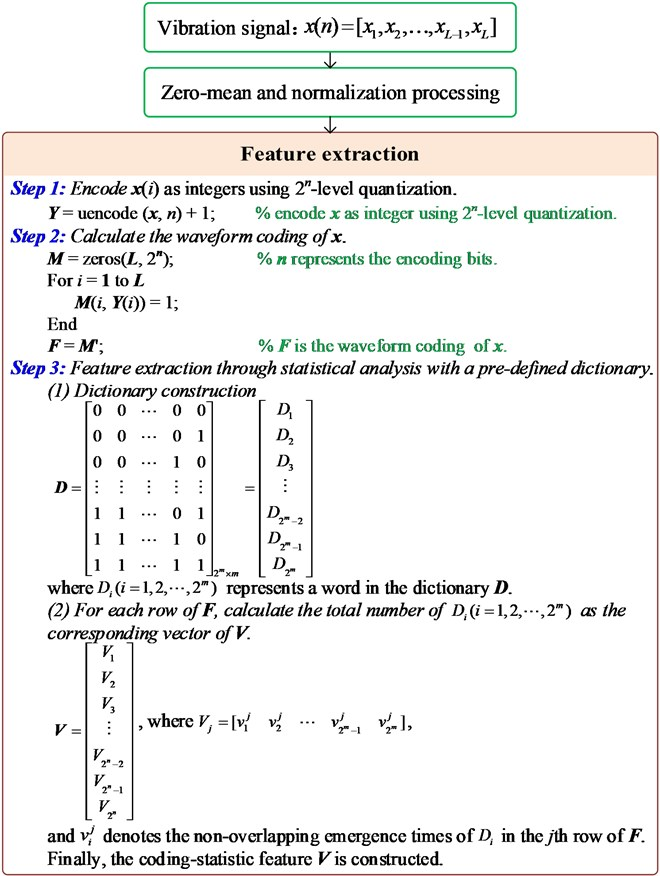Step 4: Feature extraction through statistical analysis with a pre-defined dictionary. In order to perform feature extraction with the WCM of $x$, a dictionary $\mathbit{D}$ is predefined as Eq. (3) at the very start:

(3)
$\mathbf{D}={\left[\begin{array}{lllll}0& 0& \cdots & 0& 0\\ 0& 0& \cdots & 0& 1\\ 0& 0& \cdots & 1& 0\\ ⋮& ⋮& ⋮& ⋮& ⋮\\ 1& 1& \cdots & 0& 1\\ 1& 1& \cdots & 1& 0\\ 1& 1& \cdots & 1& 1\end{array}\right]}_{{2}^{m}×m}=\left[\begin{array}{c}{D}_{1}\\ {D}_{2}\\ {D}_{3}\\ \\ {D}_{{2}^{m}-2}\\ {D}_{{2}^{m}-1}\\ {D}_{{2}^{m}}\end{array}\right],$

where stands for a word in dictionary $\mathbf{D}$ with length $m$. Afterward, the total number of each word ${D}_{i}$ in every row of $\mathbf{F}$ is calculated, and then arranged in a statistical matrix , each element of which denotes the non-overlapping emergence times of ${D}_{i}$. A coding-statistic feature $\mathbf{V}$ is constructed through the statistical analysis of $\mathbf{F}$ with the pre-defined dictionary $\mathbf{D}$ in the end.

The detailed step descriptions are presented in Fig. 1. As illustrated in Fig. 1, the calculation efficiency of the proposed coding form can be highlighted due to its omitting for the repetitive segments, especially for real-time condition monitoring system.

Next, the online computing process of the WCM is depicted with a simple parameter setting and the statistical feature extraction is illustrated intuitively. Fig. 2 gives an example of real-time waveform coding in condition monitoring system. Here, the sample length is set to $L=$ 18, and the quantization parameter is set to $n=$ 4.

Fig. 2. An example of online waveform coding in condition monitoring system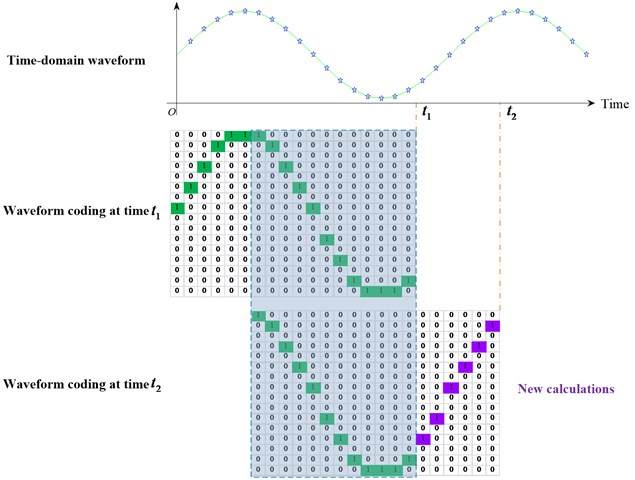In the beginning, the waveform coding of signal acquired at time ${t}_{1}$ is performed to obtain the first WCM. While collecting the second coding sample at time ${t}_{2}$, these parts marked with grey box, which belong to repetitive calculations, cannot be encoded to reduce computational burden. Hence, only those parts whose elements are marked as pink, are calculated via waveform coding algorithm. Eventually the second waveform coding is obtained by combining the repeated coding ones with the new coding ones. From the computing process, it can be seen that the computation complexity is reduced thanks to this manipulation.

In the stage of statistical feature extraction, for simplicity, the length of word in pre-defined dictionary is set to $m=$ 3. Then the dictionary $\mathbf{D}$ is determined as follows:

(4)
$\mathbf{D}={\left[\begin{array}{lll}0& 0& 0\\ 0& 0& 1\\ 0& 1& 0\\ 0& 1& 1\\ 1& 0& 0\\ 1& 0& 1\\ 1& 1& 0\\ 1& 1& 1\end{array}\right]}_{{2}^{3}×3}=\left[\begin{array}{l}{D}_{1}\\ {D}_{2}\\ {D}_{3}\\ {D}_{4}\\ {D}_{5}\\ {D}_{6}\\ {D}_{7}\\ {D}_{8}\end{array}\right],$

where ${D}_{1}$${D}_{8}$ denote the words in dictionary $\mathbf{D}$.

To illustrate the generating process of the statistical feature intuitively, the feature extraction process is presented in detail using the WCM at time ${t}_{1}$ shown in Fig. 2. The WCM at time ${t}_{1}$ is denoted as follows:

(5)
$\mathbf{F}=\left[\begin{array}{llllllllllllllllll}0& 0& 0& 0& 1& 1& 1& 0& 0& 0& 0& 0& 0& 0& 0& 0& 0& 0\\ 0& 0& 0& 1& 0& 0& 0& 1& 0& 0& 0& 0& 0& 0& 0& 0& 0& 0\\ 0& 0& 0& 0& 0& 0& 0& 0& 0& 0& 0& 0& 0& 0& 0& 0& 0& 0\\ 0& 0& 1& 0& 0& 0& 0& 0& 1& 0& 0& 0& 0& 0& 0& 0& 0& 0\\ 0& 0& 0& 0& 0& 0& 0& 0& 0& 0& 0& 0& 0& 0& 0& 0& 0& 0\\ 0& 1& 0& 0& 0& 0& 0& 0& 0& 1& 0& 0& 0& 0& 0& 0& 0& 0\\ 0& 0& 0& 0& 0& 0& 0& 0& 0& 0& 0& 0& 0& 0& 0& 0& 0& 0\\ 1& 0& 0& 0& 0& 0& 0& 0& 0& 0& 1& 0& 0& 0& 0& 0& 0& 0\\ 0& 0& 0& 0& 0& 0& 0& 0& 0& 0& 0& 0& 0& 0& 0& 0& 0& 0\\ 0& 0& 0& 0& 0& 0& 0& 0& 0& 0& 0& 0& 0& 0& 0& 0& 0& 0\\ 0& 0& 0& 0& 0& 0& 0& 0& 0& 0& 0& 1& 0& 0& 0& 0& 0& 0\\ 0& 0& 0& 0& 0& 0& 0& 0& 0& 0& 0& 0& 0& 0& 0& 0& 0& 0\\ 0& 0& 0& 0& 0& 0& 0& 0& 0& 0& 0& 0& 1& 0& 0& 0& 0& 0\\ 0& 0& 0& 0& 0& 0& 0& 0& 0& 0& 0& 0& 0& 0& 0& 0& 0& 0\\ 0& 0& 0& 0& 0& 0& 0& 0& 0& 0& 0& 0& 0& 1& 0& 0& 0& 1\\ 0& 0& 0& 0& 0& 0& 0& 0& 0& 0& 0& 0& 0& 0& 1& 1& 1& 0\end{array}\right]$.

For example, the calculating process of the first row of $\mathbf{F}$ in Eq. (5) can be denoted as:

(6)
${F}_{1}=\left[\begin{array}{llllllllllllllllll}0& 0& 0& 0& 1& 1& 1& 0& 0& 0& 0& 0& 0& 0& 0& 0& 0& 0\end{array}\right].$

Then the feature extraction can be performed with the dictionary defined in Eq. (4). The calculating process of the feature for ${F}_{1}$ is illustrated in Fig. 3.

Fig. 3. The feature extracting process of the 1st row of $\mathbf{F}$ (i.e. ${F}_{1}$)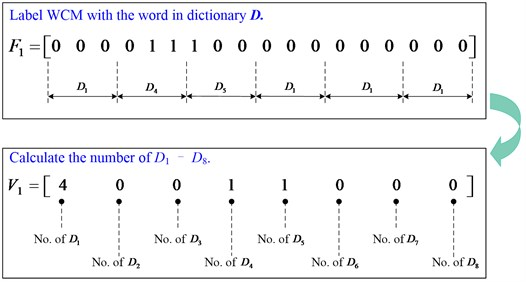Finally, with the words ${D}_{1}$${D}_{8}$ in dictionary $\mathbf{D}$, statistical feature $\mathbf{V}$ at time ${t}_{1}$ can be obtained using counting for certain word occurrence on each row of $\mathbf{F}$ presented in Eq. (5). The calculated feature $\mathbf{V}$ is shown in Eq. (7).

From the calculation process of feature extraction, it can be emphasized that the length of the sliding window ($L$) can be assigned to a larger size to contain more structure information about the analyzed object in real applications. Nevertheless, a high computational efficiency will be still retained for condition monitoring:

(7)
$\mathbit{V}=\left[\begin{array}{llllllll}4& 0& 0& 1& 1& 0& 0& 0\\ 4& 0& 1& 0& 1& 0& 0& 0\\ 6& 0& 0& 0& 0& 0& 0& 0\\ 4& 2& 0& 0& 0& 0& 0& 0\\ 6& 0& 0& 0& 0& 0& 0& 0\\ 4& 0& 1& 0& 1& 0& 0& 0\\ 6& 0& 0& 0& 0& 0& 0& 0\\ 4& 0& 1& 0& 1& 0& 0& 0\\ 6& 0& 0& 0& 0& 0& 0& 0\\ 6& 0& 0& 0& 0& 0& 0& 0\\ 5& 1& 0& 0& 0& 0& 0& 0\\ 6& 0& 0& 0& 0& 0& 0& 0\\ 5& 0& 0& 0& 1& 0& 0& 0\\ 6& 0& 0& 0& 0& 0& 0& 0\\ 4& 1& 1& 0& 0& 0& 0& 0\\ 4& 1& 0& 0& 0& 0& 1& 0\end{array}\right].$

In subsequent section, the fault diagnosis scheme using the derived statistical features will be presented combined with 2DPCA and NNC.

#### 3.1. A description of 2DPCA

Two-dimensional principal component analysis (2DPCA), as a powerful tool for processing two-dimension data, was developed for image representation , and recently was found to be used in fault diagnosis of rotating machinery . In 2DPCA, the global scatter matrix is constructed and analyzed, and usually its largest partial eigenvalues are employed to calculate the projection base. Finally, all original features are projected into eigenspace to obtain the dimension-decreased information (or called sensitive features). The principle of 2DPCA can be concluded as follows.

Suppose that there are $M$ two-dimensional samples, and the sample is denoted as ${A}_{j}$, $\left(j=1,2,\cdots ,M\right)$ herein ${A}_{j}\in {R}^{w×h}$. The global scatter matrix $G$ is first computed as:

(8)
$G=\frac{1}{M}{\sum }_{j=1}^{M}\left({A}_{j}-\stackrel{-}{A}{\right)}^{T}\left({A}_{j}-\stackrel{-}{A}\right)\in {R}^{h×h},$

where $\overline{A}=\frac{1}{M}\sum _{j=1}^{M}{A}_{j}$ is the mean matrix, and represents the transposition operation.

Then the eigenvalues and eigenvectors of $G$ is derived by solving:

(9)
$Gu=\lambda u.$

Sorting in decreasing order, the eigenvalues can be denoted as:

(10)
${\lambda }_{\mathrm{m}\mathrm{a}\mathrm{x}}^{1}\ge {\lambda }_{\mathrm{m}\mathrm{a}\mathrm{x}}^{1}\ge \cdots \ge {\lambda }_{\mathrm{m}\mathrm{a}\mathrm{x}}^{h},$

and the eigenvectors can be denoted according to Eq. (10) as:

(11)
$\mathbf{u}=\left\{\begin{array}{llll}{u}_{\mathrm{m}\mathrm{a}\mathrm{x}}^{1}& {u}_{\mathrm{m}\mathrm{a}\mathrm{x}}^{2}& \cdots & {u}_{\mathrm{m}\mathrm{a}\mathrm{x}}^{h}\end{array}\right\}.$

Before determining the optimal projection axes to be applied to perform dimensionality reduction, an eigenvector selecting criterion is introduced here:

(12)
$\frac{\sum _{j=1}^{d}{\lambda }_{\mathrm{m}\mathrm{a}\mathrm{x}}^{j}}{\sum _{j=1}^{h}{\lambda }_{\mathrm{m}\mathrm{a}\mathrm{x}}^{j}}\ge \gamma ,$

where $\gamma$ is the selecting threshold, and it is specified as $\gamma =$ 90 % in this study.

According to Eq. (12), the optimal projection coordinate can be constructed as below:

(13)
$\mathbf{U}=\left\{\begin{array}{llll}{u}_{\mathrm{m}\mathrm{a}\mathrm{x}}^{1}& {u}_{\mathrm{m}\mathrm{a}\mathrm{x}}^{2}& \cdots & {u}_{\mathrm{m}\mathrm{a}\mathrm{x}}^{d}\end{array}\right\}.$

For a given sample $\mathbf{B}\in {R}^{w×h}$, it can be transformed with the above derived coordinate as:

(14)
$\mathbf{Y}=\mathbf{B}\mathbf{U}\in {R}^{w×d}.$

Finally, the eigen matrix of $\mathbf{B}$ can be evaluated as $\mathbf{Y}$. More detailed descriptions about 2DPCA can be discovered in Ref. .

#### 3.2. NNC-based classification

After reducing the dimension of the features, an NNC, a nearest Frobenius distance classifier, is applied to construct the state recognition model. Here, the distance between a projected test sample, $\mathbf{T}\in {R}^{w×d}$ and a projected base sample $\mathbf{E}\in {R}^{w×d}$, is measured with Frobenius norm as:

(15)
$d\left(\mathbf{T},\mathbf{E}\right)={‖\mathbf{T}-\mathbf{E}‖}_{F},$

where ${‖•‖}_{F}$ denotes the Frobenius norm.

As mentioned above, the optimal projection coordinate is obtained as shown in Eq. (13). Therefore the $M$ training samples can be projected into eigen space and denoted as ${E}_{1},{E}_{2},\cdots ,{E}_{M}$. Finally, given a testing sample $T$, if:

(16)
$d\left(\mathbf{T},{\mathbf{E}}_{r}\right)=\underset{j}{\mathrm{min}}d\left(\mathbf{T},{\mathbf{E}}_{j}\right),$

and ${\mathbf{E}}_{r}$ belongs to the class identity ${\omega }_{m}$ (where $m\in \left\{1,2,\cdots ,{N}_{class}\right\}$ and ${N}_{class}$ is the total class number of the training samples), the resulting decision is that $\mathbf{T}$ is classified as ${\omega }_{m}$.

After this, the real-time collected vibration signal features are also assessed with the projection coordinate that obtained in the NNC training phase, and then input into the state recognition model to identify the bearing health state.

#### 3.3. The proposed scheme for bearing diagnosis

The framework of the proposed scheme for bearing diagnosis is depicted in Fig. 4.

The proposed method is decomposed into two main steps. The first step is done offline and aims at generating a classification model. The second step, which is achieved online, utilizes the model generated in the first step to classify the bearing health state. The process of the proposed method for offline training is given below:

(1) Vibration signal is first measured from the bearing system using acceleration sensors.

(2) Divide the original signal into equal time segments with a sliding window (length: $L$) and M sub-signals are obtained.

(3) Perform feature extraction according to the methodology described in section 2.

(4) Reduce dimensionality and remove redundancy using 2DPCA, and obtain the projection coordinate $U$.

(5) Train NNC model by projecting all training samples with $\mathbf{U}$ into eigen space to obtain the training projective features ${E}_{j}$ (1, 2,…, $M$).

For online fault diagnosis, the testing vibration signal is also acquired with length $L$, and the same process of WCM-based feature extraction is conducted to derive the original feature. Finally, it is input into the NNC model to decide its current state. In addition, the computation complexity of the proposed method could be less than the traditional fault diagnosis methods, due to the WCM is derived by a slipping scheme in feature extraction phase when applied in online monitoring.

Fig. 4. Framework of the proposed diagnostic scheme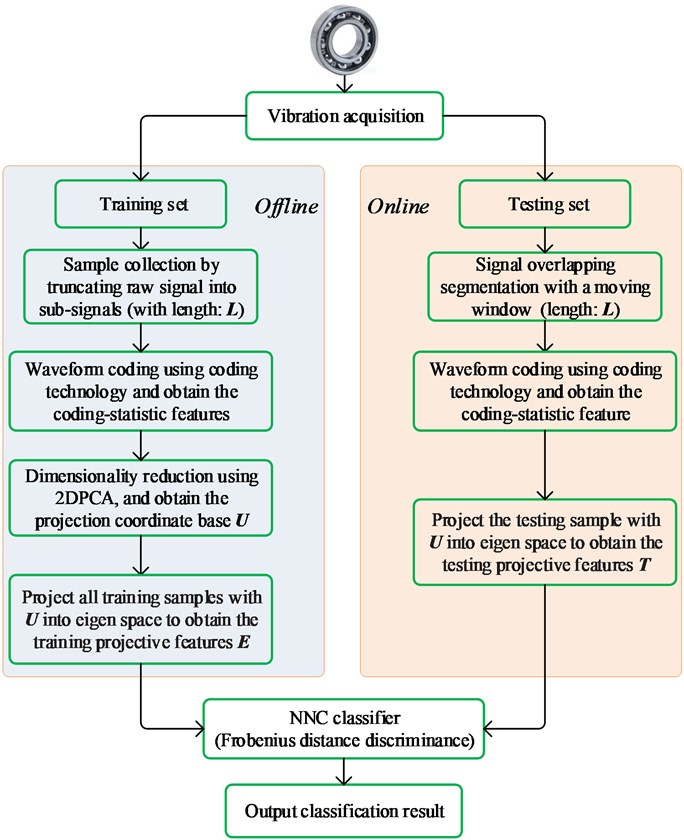#### 4.1. Simulation study

To express the structures of the collected signals using the proposed coding technology more intuitively, a simulation presentation was conducted first. In this subsection, an impulse signal is employed to observe the performance of the waveform coding. An modified impulse signal from Ref.  is expressed in Eq. (17):

(17)

where ${f}_{m}$, $f$ are equal to 110 and 3,900 respectively, the uniformly distributed random number is used to simulate the randomness caused by the slippage, ${F}_{s}$ is the sampling frequency (${F}_{s}=$ 12,000 Hz). And $\left(k-r×{F}_{s}/{f}_{m}-{\tau }_{r}\right)/{F}_{s}\right)\ge 0$ is applied to ensure the causality of the exponential function.

Fig. 5. Impulse signal: a) time waveform; b) WCM of a)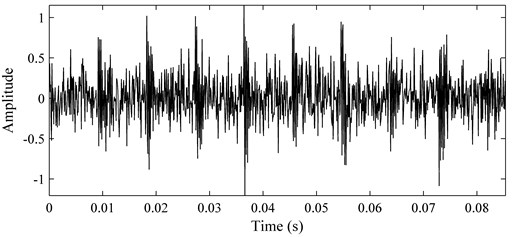a)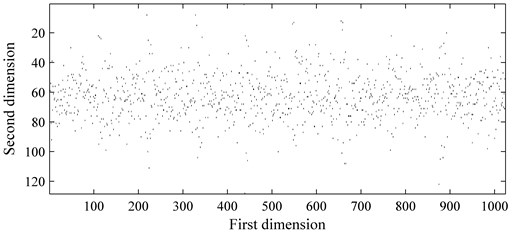b)

In this chapter, the presence of additive Gaussian noise for the simulated signals are considered, and the signal noise ratio (SNR) is set as –2 dB. This simulation study aims to show the waveform coding process of the proposed method. According to the generation process of WCM, it can be seen that the WCM can be dug as the eigenstate of the simulated signals. It is clear that, the intrinsic structure of the analyzed signal is exploited into a two-dimensional matrix. The original signal and its WCM are shown in Fig. 5, where only 1024 sampling points are displayed.

The feature extraction of the simulated data is omitted on account of the large length of the signals (i.e., $L=$ 1024), with which the feature matrix $\mathbf{V}$ is too large to demonstrate here. For the technical details about feature extraction, readers can refer to the example presented in Fig. 3.

Fig. 6. The test rig for bearing fault diagnosis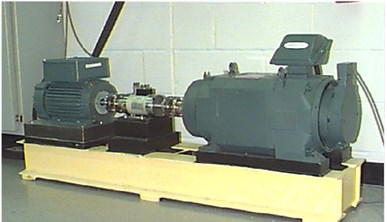#### 4.2.1. Bearing data description

In order to evaluate the performance of the proposed methodology, vibration signals collected from Case Western Reserve University  are utilized to validate the effectiveness of the diagnostic scheme. As depicted in Fig. 6, the test rig consists of a 2 hp motor (left), a torque transducer/encoder (center), a dynamometer (right), and control electronics (not shown). Bearing with fault diameters of 0.007 inches, 0.014 inches, 0.021 inches, 0.028 inches, were installed on the motor housing at the drive end of the motor to acquire the vibration signals. Vibration signals under four different load/speed conditions, i.e. C1 = 0 hp/1797 rpm, C2 = 1 hp/1772 rpm, C3 = 2 hp/1750 rpm and C4 = 3 hp/1730 rpm, were collected with a sampling frequency of 1,2000 Hz. The experiment conditions are illustrated in Table 1. The bearing data sets were obtained from the experimental bench under the four different health conditions: (1) normal condition (NO); (2) with inner race fault (IF); (3) with outer race fault (OF); and (4) with ball fault (BF). Fig. 7 illustrates the WCMs obtained from bearings under different health status in C3 operating condition. In this work, the length of the sub-signal (or the moving window) is set as 4096, and the quantization bit n and word length m are empirically designated as 7 and 3 respectively.

Fig. 7. Time-domain waveforms and WCMs of four different operation statuses in C3 condition: a), b) normal condition; c), d) inner race fault; e), f) ball fault and g), h) outer race fault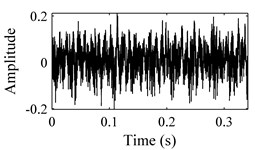a)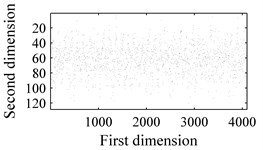b)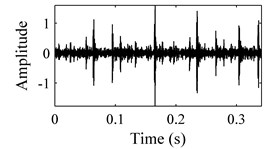c)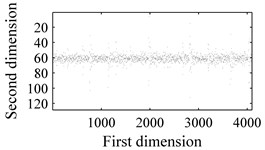d)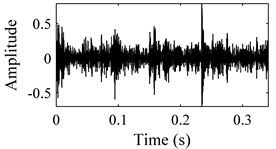e)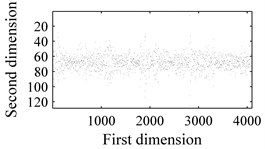f)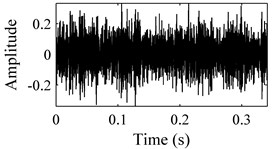g)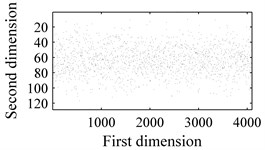g)

Table 1. Illustration of the experiment conditions

 Experiment conditions C1 C2 C3 C4 Speed (rpm) 1797 1772 1750 1730 Load (hp) 0 1 2 3

In this section, two experiments were conducted over two different data subsets (A-B) to fully verify the robustness of the proposed method. During the random segmentation phase, the samples were randomly extracted with a fix time window from the acquired raw vibration signals. And 200 samples were created for each bearing with NO (or IF, or OF and or BF) under load condition C1 (or C2, or C3 and or C4). The two classification problems are summarized in Table 2.

Table 2. Description of the bearing data sets

 Data set No. of samples (training/testing) Defect size (training/testing) Health condition Label A 400/400 0/0 Normal 1A 400/400 0.007/0.007 Ball fault 2A 400/400 0.007/0.007 Inner race fault 3A 400/400 0.007/0.007 Outer race fault 4A B 400/400 0/0 Normal 1B 400/400 0.007/0.007 Ball fault 2B 400/400 0.014/0.014 Ball fault 3B 400/400 0.021/0.021 Ball fault 4B 400/400 0.007/0.007 Inner race fault 5B 400/400 0.014/0.014 Inner race fault 6B 400/400 0.021/0.021 Inner race fault 7B 400/400 0.007/0.007 Outer race fault 8B 400/400 0.014/0.014 Outer race fault 9B 400/400 0.021/0.021 Outer race fault 10B

Table 3. Diagnosis performance using the proposed method

 Data set Testing condition Average accuracy C1 (0 hp/1797 rpm) C2 (1 hp/1772 rpm) C3 (2 hp/1750 rpm) C4 (3 hp/1730 rpm) A 94.30 % 95.36 % 95.15 % 91.88 % 94.42 % B 95.65 % 95.47 % 95.06 % 93.07 % 94.81 %

#### 4.2.2. Diagnostic performance analysis

In order to eliminate the redundancy of the original coding statistic features and improve the computational efficiency, all collected original features for training sets are tackled by means of 2DPCA algorithm to calculate the projection coordinate for reduction of dimensionality. While processing using 2DPCA, the contribution of the selected largest eigenvalues is set as $\gamma =$ 90 %, to establish the projection coordinate. Then the original training and testing features are mapped with the projection basis. As a result, the NNC is trained with the dimension-reduced features of training samples to derive the state classification model. One other thing to note is that training samples under the four load conditions are all applied to train the NNC model in the training phase, and each experimental routine is performed 20 times via randomly selecting training samples, then the average accuracies of the 20 randomized trials are calculated and recorded. The diagnostic performances of the two data sets are shown in Table 3.

Examining the diagnostic performance from Table 3, it can be observed that high accuracies are achieved for both data set A and B. For the first classification problem, testing samples from C1, C2, C3 and C4 were classified with accuracy 94.30 %, 95.36 %, 95.15 % and 91.88 % respectively. Average accuracy, 94.42 %, is obtained and it indicates that the proposed method could diagnose the health states of bearing with satisfied performance. Moreover, for the second classification problem, testing samples from C1, C2, C3 and C4 were classified with accuracy 95.65 %, 95.47 %, 95.06 % and 93.07 % respectively. This shows that the proposed method could be capable of handling this multi-class problem to perform bearing fault classification. Meanwhile, an interesting phenomenon is noticed that the average accuracy using data set B (10 class) is little higher than that using data set A (4 class). The main reason is that the samples in the class of 2B, 5B and 8B are more misclassified than others.

In order to compare the performance for fault diagnosis more intuitively, some published publications are convened to make comparisons in Table 4.

Table 4. Diagnostic performance comparison with some published works

 Method Loadcondition Classified problem (training/testing) Accuracy (%) Multiple ANFIS combination  Multiple 4 class (both 0.007) 100.00 10 class (both 0, 0.007, 0.014, 0.021) 91.33 SVR + phase space features  Single 10 class (both 0, 0.007, 0.014, 0.021) 90.30 TR-LDA2 + kNN classifier  Single 10 class (both 0, 0.007, 0.014, 0.021) 92.50-98.00 NNC + coding features [present work] Multiple 4 class (both 0.007) 94.42 10 class (both 0, 0.007, 0.014, 0.021) 94.81

Validating with the same bearing data, time- and frequency-domain features are calculated and classified with multiple adaptive neuro-fuzzy inference system (ANFIS) combination in , in which 100.00 % and 91.33 % are obtained for the two classification problems respectively. As for the ten-class classification problem, phase space features and Support Vector Regression Machine (SVR) in , trace ratio criterion LDA (TR-LDA) and kNN classifier in  are put forward to deal with this complicated situation, and accuracies of 90.30 %, 92.50-98.00 % and 99.38 % are obtained respectively. Meanwhile, the present work with 94.81 % classification accuracy seems to be another excellent diagnostic method in handling this problem. Through the comparison and analysis, it can be seen that the proposed diagnostic scheme could demonstrate excellent performance on fault classification problems, overall. Due to the excellent efficiency of feature extraction and the outstanding performance for fault classification, the proposed method is also applicable to be applied in the fault diagnosis system for industrial applications.

#### 5. Conclusions

Obviously, the waveform coding algorithm proves that the nature and regularity of the time series could be grasped deeply. More and higher requirements are being put forward for industrial applications, as well as the efficiency of the utilized algorithms is receiving more and more attentions. Based on this consideration, a WCM-based feature extraction method is proposed in this paper. At first, the statistical feature is acquired directly from WCM of the time domain series with the assistance of a coding algorithm. Then 2DPCA is employed to tackle these statistical features obtained in previous phase. Finally, fault classification with NNC is applied for classification. Two groups of experiments are conducted to validate the performance of the presented methodology. Reviewing the process of feature extraction and the effectiveness for fault classification, some conclusions are derived as follows.

1) The proposed algorithm has small algorithmic complexity and high efficiency. Thanks to its non-repetitive calculation for the overlapping sections as illustrated in Fig. 2, the proposed algorithm is well suited for online monitoring in real-time fault diagnosis systems.

2) The diagnosis scheme in this paper could show an excellent performance in handling the multi-class classification problems like data set B in Table 2.

As described in Section 2, it can be seen that the parameter $n$ for quantization and $m$ for WCM-based feature calculation may influence the performance and robustness of the proposed coding method. And they are both selected empirically. Therefore, a future work will be aimed at the optimized selection of these parameters to further improve the robustness of the proposed method.

#### Acknowledgements

This work was supported by the Natural Science Foundation of Guizhou Province (ZKYB270) and the Research Foundation of Guizhou Minzu University (GZMUQN06). The authors would also like to thank Case Western Reserve University for sharing the bearing fault data on the Internet.

1. D. Abboud, M. Elbadaoui, W. A. Smith, and R. B. Randall, “Advanced bearing diagnostics: A comparative study of two powerful approaches,” Mechanical Systems and Signal Processing, Vol. 114, pp. 604–627, Jan. 2019, https://doi.org/10.1016/j.ymssp.2018.05.011 [Publisher]
2. J. Qi, X. Gao, and N. Huang, “Mechanical fault diagnosis of a high voltage circuit breaker based on high-efficiency time-domain feature extraction with entropy features,” Entropy, Vol. 22, No. 4, p. 478, Apr. 2020, https://doi.org/10.3390/e22040478 [Publisher]
3. F. Jiang, K. Ding, G. He, and C. Du, “Sparse dictionary design based on edited cepstrum and its application in rolling bearing fault diagnosis,” Journal of Sound and Vibration, Vol. 490, p. 115704, Jan. 2021, https://doi.org/10.1016/j.jsv.2020.115704 [Publisher]
4. X. Wang, Y. Li, T. Rui, H. Zhu, and J. Fei, “Bearing fault diagnosis method based on Hilbert envelope spectrum and deep belief network,” Journal of Vibroengineering, Vol. 17, No. 3, pp. 1295–1308, May 2015. [Search CrossRef]
5. S. Zhou, M. Xiao, P. Bartos, M. Filip, and G. Geng, “Remaining useful life prediction and fault diagnosis of rolling bearings based on short-time Fourier transform and convolutional neural network,” Shock and Vibration, Vol. 2020, pp. 1–14, Oct. 2020, https://doi.org/10.1155/2020/8857307 [Publisher]
6. Y. Sun, S. Li, Y. Wang, and X. Wang, “Fault diagnosis of rolling bearing based on empirical mode decomposition and improved Manhattan distance in symmetrized dot pattern image,” Mechanical Systems and Signal Processing, Vol. 159, p. 107817, Oct. 2021, https://doi.org/10.1016/j.ymssp.2021.107817 [Publisher]
7. H. Li, Q. Zhang, X. Qin, and Y. Sun, “K-SVD-based WVD enhancement algorithm for planetary gearbox fault diagnosis under a CNN framework,” Measurement Science and Technology, Vol. 31, No. 2, p. 025003, Feb. 2020, https://doi.org/10.1088/1361-6501/ab4488 [Publisher]
8. M. Jalayer, C. Orsenigo, and C. Vercellis, “Fault detection and diagnosis for rotating machinery: A model based on convolutional LSTM, Fast Fourier and continuous wavelet transforms,” Computers in Industry, Vol. 125, p. 103378, Feb. 2021, https://doi.org/10.1016/j.compind.2020.103378 [Publisher]
9. H. Shao, J. Lin, L. Zhang, and M. Wei, “Compound fault diagnosis for a rolling bearing using adaptive DTCWPT with higher order spectra,” Quality Engineering, Vol. 32, No. 3, pp. 342–353, Jul. 2020, https://doi.org/10.1080/08982112.2020.1749654 [Publisher]
10. X. Liu, L. Bo, and H. Luo, “Bearing faults diagnostics based on hybrid LS-SVM and EMD method,” Measurement, Vol. 59, pp. 145–166, Jan. 2015, https://doi.org/10.1016/j.measurement.2014.09.037 [Publisher]
11. J. Yang, Y. Zhang, and Y. Zhu, “Intelligent fault diagnosis of rolling element bearing based on SVMs and fractal dimension,” Mechanical Systems and Signal Processing, Vol. 21, No. 5, pp. 2012–2024, Jul. 2007, https://doi.org/10.1016/j.ymssp.2006.10.005 [Publisher]
12. B. Sreejith, A. K. Verma, and A. Srividya, “Fault diagnosis of rolling element bearing using time-domain features and neural networks,” in 2008 IEEE Region 10 and the Third international Conference on Industrial and Information Systems (ICIIS), No. 1, pp. 1–6, Dec. 2008, https://doi.org/10.1109/iciinfs.2008.4798444 [Publisher]
13. D.-T. Hoang and H.-J. Kang, “A survey on deep learning based bearing fault diagnosis,” Neurocomputing, Vol. 335, pp. 327–335, Mar. 2019, https://doi.org/10.1016/j.neucom.2018.06.078 [Publisher]
14. A. Choudhary, T. Mian, and S. Fatima, “Convolutional neural network based bearing fault diagnosis of rotating machine using thermal images,” Measurement, Vol. 176, p. 109196, May 2021, https://doi.org/10.1016/j.measurement.2021.109196 [Publisher]
15. Jian Yang, D. Zhang, A. F. Frangi, and Jing-Yu Yang, “Two-dimensional PCA: a new approach to appearance-based face representation and recognition,” IEEE Transactions on Pattern Analysis and Machine Intelligence, Vol. 26, No. 1, pp. 131–137, Jan. 2004, https://doi.org/10.1109/tpami.2004.1261097 [Publisher]
16. W. Li, M. Qiu, Z. Zhu, B. Wu, and G. Zhou, “Bearing fault diagnosis based on spectrum images of vibration signals,” Measurement Science and Technology, Vol. 27, No. 3, p. 035005, Mar. 2016, https://doi.org/10.1088/0957-0233/27/3/035005 [Publisher]
17. W. Li, M. Qiu, Z. Zhu, F. Jiang, and G. Zhou, “Fault diagnosis of rolling element bearings with a spectrum searching method,” Measurement Science and Technology, Vol. 28, No. 9, p. 095008, Sep. 2017, https://doi.org/10.1088/1361-6501/aa7b4c [Publisher]
18. Bearings Vibration Dataset, Case Western Reserve University, 2003. [Search CrossRef]
19. Y. Lei, Z. He, Y. Zi, and Q. Hu, “Fault diagnosis of rotating machinery based on multiple ANFIS combination with GAs,” Mechanical Systems and Signal Processing, Vol. 21, No. 5, pp. 2280–2294, Jul. 2007, https://doi.org/10.1016/j.ymssp.2006.11.003 [Publisher]
20. F. Liu, B. He, Y. Liu, S. Lu, Y. Zhao, and J. Zhao, “Phase space similarity as a signature for rolling bearing fault diagnosis and remaining useful life estimation,” Shock and Vibration, Vol. 2016, pp. 1–12, 2016, https://doi.org/10.1155/2016/5341970 [Publisher]
21. M. Zhao, X. Jin, Z. Zhang, and B. Li, “Fault diagnosis of rolling element bearings via discriminative subspace learning: visualization and classification,” Expert Systems with Applications, Vol. 41, No. 7, pp. 3391–3401, Jun. 2014, https://doi.org/10.1016/j.eswa.2013.11.026 [Publisher]

#### Cited By

 Applied Sciences Jing Wang, Mengli Zhao, Xiao Xie, Li Zhang, Wenbo Zhu 2022